# Electronics and Communication Engineering - Exam Questions Papers

26.

Figure shows four D type FFs are connected as a shift register using an XOR gate. The initial state and 3 subsequent states for 3 clock pulses are also given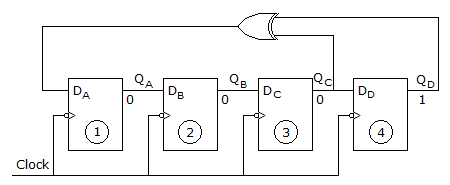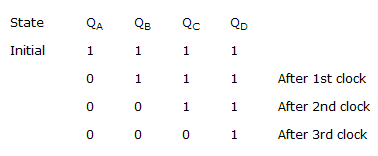The state QA QB QC QD after the 4th clock pulse is

 A. 0000 B. 1111 C. 1001 D. 1000

Explanation:

After the 3rd pulse FF3 is 0 and FF4 is 1, so that XOR output is 1 which is fed to DA.

So, QA = 1, QA to QB → 0

AB to AC → 0, QC → QD → 0.

27.

A rectangular wave guide is designed to propagate at the dominant mode TE10 at a frequency of 5 GHz. The cut-off frequency is 0.8 of signal frequency. The ratio of the guide width to height is 2. The dimensions of the guide are

 A. 3.75 cm, 1.875 cm B. 4 cm, 2 cm C. 8 cm, 4 cm D. 2.54 cm, 1.27 cm

Explanation:

λc = 2a or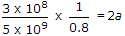a = 3.75 cm

Also,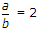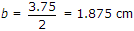.

28.

In the Taylor series expansion of exp(x) + sin(x) about the point x = p, the coefficient of (x - p)2 is

 A. exp (p) B. 0.5 exp (p) C. exp (p) + 1 D. exp (p) - 1

Explanation:

f(x) = ex + sin x

Coefficient of (x - p)2 = 1 / 2! f''(x)

f'(x) = ex + cos x

f'(x) = ex - sin x

f''(x)|x=p = ep

Thus coefficient of (x - p)2 = 0.5 .

29.

The Z transform of a particular signal is given as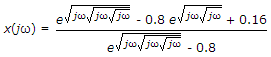The practical implementation of the system will be

 A. non causal and stable B. causal and stable C. unstable and noncausal D. can't say

Explanation: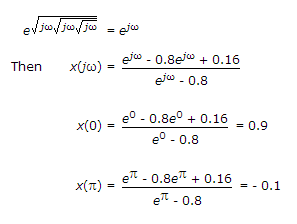When get high value at ω = 0, and low value at ω = p, hence we get a low pass filter.

So the system is min-phase causal system. For practical implementation of the system, the system has to be stable.

30.

If g(t) = e-pt2 then G(1/p) is __________ where g(t)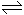G(f)

 A. e-2/p B. e-1/p C. e-p D. e2p

Explanation:

The signal g(t) given above is a gaussian pulse and it satisfies the relation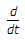g(t) = - 2pt g(t)

Its fourier transform is same as the signal itself in frequency domain

G(f) = e-pf2

G(1/p) = e-p(1/p)2 = e-1/p.

#### Current Affairs 2022

Interview Questions and Answers